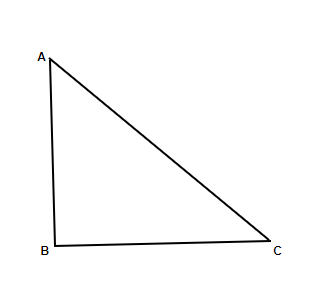# If tan θ = 2/3, then sin θ = ?

• Last Updated : 12 Oct, 2021

Trigonometry is a very important branch of Mathematics. The famous Greek Astronomer and mathematician Hipparchus was the inventor of this special branch of mathematics. The name ‘Trigonometry’ implies that the method of measurement relative to triangles is the subject of trigonometry (note that ‘trigonon’ means triangle and ‘metron’ means measurement method). To put it clearly, the special branch of mathematics that deals with three sides of a triangle, its three angles, its area, and their interrelationships is called Trigonometry.Attention reader! All those who say programming isn't for kids, just haven't met the right mentors yet. Join the  Demo Class for First Step to Coding Coursespecifically designed for students of class 8 to 12.

The students will get to learn more about the world of programming in these free classes which will definitely help them in making a wise career choice in the future.

In this right-angled triangle, let the reference angle between base and hypotenuse is θ (theta). On the longest side, the hypotenuse is directly opposite to the right angle, which is also the largest angle among the three angles.

In a right-angle triangle, sinθ = perpendicular / hypotenuse; cosθ = base / hypotenuse ; tanθ = perpendicular / base

Example, tan A = (perpendicular / hypotenuse) / (base / hypotenuse)

= sin A / cos A , where A is any angle without 90° in right angle triangle.

Some extra trigonometric rules,

cotθ = 1 / tanθ = base / perpendicular

secθ = 1 / cosθ = hypotenuse / base

cosecθ = 1 / sinθ = hypotenuse / perpendicular, where θ is any angle without 90°

### Trigonometric Angles

The values of trigonometric expectant of any angle are either positive or negative. From the definitions of trigonometric expectant, in this picture,

sin A = BC/AB and cos A = CA/AB

Now, AB is the hypotenuse of the right-angled triangle ABC. Thus, the value of AB is always greater than BC & CA. So, the value of sin A and cos A always be less than or equal to 1.

then, cosec A = AB/BC and sec A = AB/CA

So, the value of cosec A and sec A always be greater than or equal to 1

Also, tan A = BC/CA and cot A = CA/BC

Clearly, the value of BC can be either larger or smaller than CA. So, In a right-angled triangle, the hypotenuse is the largest side among them. θ is a positive angle, then:

1. The value of sinθ, cosθ cannot be greater than 1.

2. The value of cosecθ, secθ cannot be less than 1.

3. Tanθ, cotθ have any value.

#### Some Important formulae

• sin2A + cos2A = 1
• sec2A – tan2A = 1 and,
• cosec2A – cot2A = 1

### If tan θ = 2/3, then sin θ =?

Solution:

Now, If tan A = 2/3, cot A = 3/2

Then, cosec2A = 1 + cot2A  [from 3rd rule]

= 1 + (3/2)2

= 1 + 9/4

= 13/4

So, cosec A = √(13/4) = √13/2

So, sin A = 1 / cosec A = 2/√13

sin A = 2 / √13.

### Similar Problems

Question 1: If tanθ = -1/√5, then cosθ = ?

Solution:

tanθ = -1/√5

Then, sec2θ = 1 + tan2θ

= 1 + (-1/√5)2

= 1 + (1/5)

⇒ sec2θ = 6/5

⇒ secθ = √(6/5)

So, cosθ = 1 / secθ = √(5/6)

cosθ = √(5/6)

Question 2: If cosecφ = 2/√3, then φ = ?

Solution:

cosecφ = 2/√3

⇒  φ = cosec-1(2/√3) = 60°

φ = 60°

Question 3: If tanα = 5/12 and sinα is -ve(negative), then cosα = ?

Solution:

tanα = 5/12

Then, sec2α = 1 +tan2α

= 1 + (5/12)2

= 1 + (25/144)

⇒ sec2α = 169/144

⇒ secα = √(169/144) = ±(13/12)

In this question, sinα = -ve (negative) (given) and tanα is positive, so cosα is obviously negative

Then, cosα = -12/13

cosα = -12/13

My Personal Notes arrow_drop_up